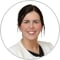IFRS

# Insights into IFRS 16: Understanding the discount rateLouise Kelly

Under IFRS 16 ‘Leases’, discount rates are used to determine the present value of the lease payments used to measure a lessee’s lease liability. Discount rates are also used to determine lease classification for a lessor and to measure a lessor’s net investment in a lease.

For lessees, the lease payments are required to be discounted using:

• the interest rate implicit in the lease (IRIL), if that rate can be readily determined, or
• the lessee’s incremental borrowing rate (IBR).

For lessors, the discount rate will always be the interest rate implicit in the lease.

The interest rate implicit in the lease is defined in IFRS 16 as ‘the rate of interest that causes the present value of (a) the lease payments and (b) the unguaranteed residual value to equal the sum of (i) the fair value of the underlying asset and (ii) any initial direct costs of the lessor.’

The lessee’s incremental borrowing rate is defined in IFRS 16 as ‘the rate of interest that a lessee would have to pay to borrow over a similar term, and with a similar security, the funds necessary to obtain an asset of a similar value to the right-of-use asset in a similar economic environment’.

The incremental borrowing rate is determined on the commencement date of the lease. As a result, it will incorporate the impact of significant economic events and other changes in circumstances arising between lease inception and commencement.

A lessee will need to determine a discount rate for virtually every lease to which it applies the lessee accounting model in IFRS 16. However, a discount rate may not need to be determined for a lease if:

• a lessee applies the recognition exemption for either a shortterm or a low-value asset lease
• all lease payments are made on (or prior to) the commencement date of the lease, or
• all lease payments are variable and not dependent on an index or rate (eg, all lease payments vary based on sales or usage).

The interest rate implicit in the lease may be similar to the lessee’s incremental borrowing rate in many cases. Both rates consider the credit risk of the lessee, the term of the lease, the security and the economic environment in which the transaction occurs.